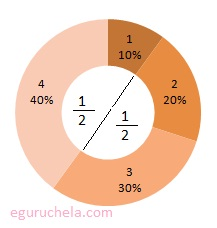# Fraction Operations

 A C + = + = B D

 A C - = - = B D

When adding or subtracting fractions, we ensure that the fractions being added have the same denominator.

If they do not, find the LCD for the fractions and put each in its equivalent form.

Then, simply add or subtract the numerators of the fractions.

 A C x = x = B D

 A C ÷ = ÷ = B D

When multiplying fractions, we simply multiply the numerators together and then multiply the denominators together.

When you divide two fractions, we take the reciprocal of the second fraction or bottom fraction, and multiply.

A fraction is a number that represents a part of a whole and consists of a numerator and a denominator. The numerator represents the number of equal parts of the whole and the denominator is the total number of parts that are said to be whole number. For example: 1/2 , the numerator is 1 and the denominator is 2.
Another example include pie with 4 slices. Every slices will constitute a fraction and the total of 4 slices that comprises the whole pie means 10 equale size of slices (4+1+2+3 = 10). If someone hold P1 (that is 4) and P2 (that is 1) slices, the remaining portion of the pie would be 10 - 5 = 5/10 slices which is 1/2 in fraction or 50%.